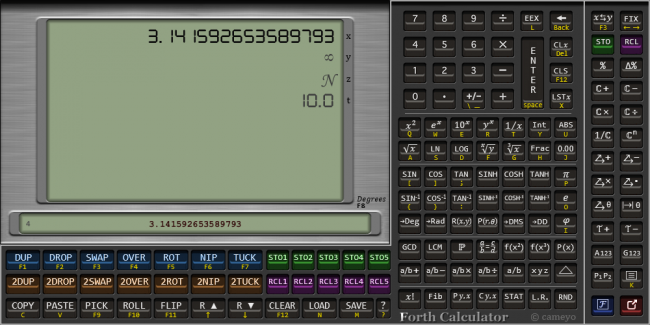Howdy, Stranger!

We are about to switch to a new forum software. Until then we have removed the registration on this forum.

Forth Calculator (RPN) final version

edited November 2017

I am learning Forth with "Starting Forth" and "Thinking in Forth" books by Leo Brodie.
I need to visualize how works the stack...and then write a processing sketch.Features:
Scientific Calculator (RPN/Forth)
Features:
- 75 mathematical functions
- 8 cells display
- 4096 cells stack
- 5 memories
- Forth words to manage the stack (DUP, DROP, SWAP, ecc.)
- GDC, MCM, Factorize number
- Solve proportion
- Solve Linear System
- Solve quadratic and cubic equation (real and complex roots)
- Complex arithmetic
- Vector arithmetic
- Fraction arithmetic
- Polar Coord <-> Rect coord
- Number to Fraction Generator
- Combinations, Permutations
- Factorial, Fibonacci (BigInteger)
- Degrees Minutes Seconds <-> Decimal Degrees
- Sequence generator
- Statistical parameters of a serie
- Linear Regression
- Slope
- Time arithmetic
- Copy and Paste stack numbers
- Save and Load stack numbers
- Edit stack numbers with external text editor
- Infinity and NaN numbers
- Italian and English manual (60 pages)
- ...
Fast Fibonacci routine
https://www.nayuki.io/page/fast-fibonacci-algorithms

Press 'TAB' to see shortcuts.
Hints and bugs report are welcome.
cameyo

Tagged:

• Are you trying to create a Forth emulator?

• No. It is only a calculator with an unlimited (almost) stack manipulable with Forth WORDS (dup, drop,...).

• New functions:
- complex arithmetic
- time arithmetic
I'm working to solve some small bugs :)

• The sketch is complete :)
I use it in my daily work.
Thanks for watching.
cameyo